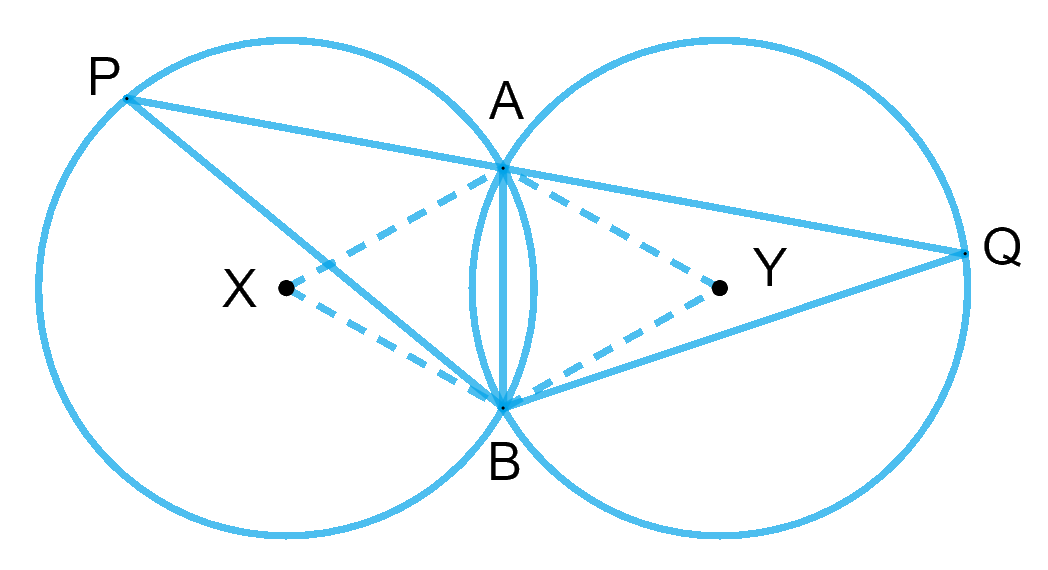# Ex.10.6 Q9 Circles Solution - NCERT Maths Class 9

Go back to  'Ex.10.6'

## Question

Two congruent circles intersect each other at points \begin{align}{A} \end {align} and \begin{align}{B.}\end {align} Through \begin{align}{A} \end {align} any line segment \begin{align}{PAQ} \end {align} is drawn so that \begin{align}{P, Q} \end {align} lie on the two circles. Prove that \begin{align}{BP = BQ.} \end {align}

Video Solution
Circles
Ex 10.6 | Question 9

## Text Solution

What is known?

Two congruent circles intersect each other at points \begin{align}{A} \end {align} and \begin{align}{B.} \end {align}

To prove:

\begin{align}{BP = BQ.} \end {align}

Reasoning:

The angle subtended by an arc at the centre is double the angle subtended by it at any point on the remaining part of the circle.

Steps:\begin{align}{AB} \end {align} is the common chord to both circles. Let us join A and B to the centres $$X$$ and $$Y$$ of the respective circles.

As we know that, the angle subtended by an arc at the centre is double the angle subtended by it at any point on the remaining part of the circle.

Therefore,

\begin{align} \angle AXB &= 2\angle APB \\ \angle AYB &= 2\angle AQB \\ \end{align}

Since the circles are congruent and $$AB$$ is a common chord.

Hence, $$\Delta AXB \cong \Delta AYB$$ by SSS criteria of congruency.

$$\angle AXB = \angle AYB$$ by CPCT

Therefore, $$\angle APB = \angle AQB$$

Now, consider the $$ΔBPQ,$$

$\angle QPB = \angle PQB$

This implies that $$ΔBPQ$$ is an isosceles triangle as base angles are equal.

Therefore, we get $$BP = BQ.$$

Video Solution
Circles
Ex 10.6 | Question 9

Learn from the best math teachers and top your exams

• Live one on one classroom and doubt clearing
• Practice worksheets in and after class for conceptual clarity
• Personalized curriculum to keep up with school